ISSN: 1070-4698

# Vol. 38

Latest Volume
All Volumes
All Issues

PIER Vol. 38, 311-331, 2002. doi:10.2528/PIER02032501

###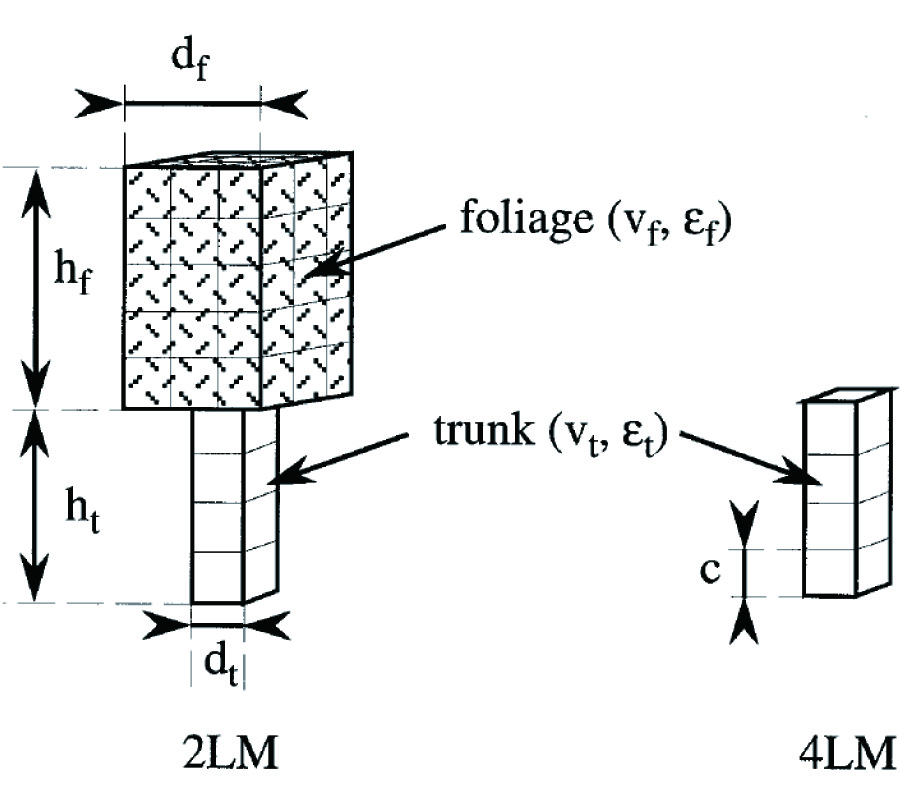A Full Wave Three Dimensional Analysis of Forest Remote Sensing Using VHF Electromagnetic Wave

Helene Roussel and Walid Tabbara

A forest made of an infinite biperiodic array of trees over a lossy ground, is illuminated by a linearly polarized electromagnetic plane wave in the range of 20 to 90 MHz. Due to the ratio of the wavelength to the array period, only the specular mode is propagative. Therefore, a reflection coefficient is computed and not a backscattering coefficient. It is obtained by means of a full wave approach, based on an integral representation of the electric field. This approach takes into account all possible interactions between each component of the medium as well as ground penetration and provides full information on the phase of the scattered field. Two models of the forest are developed, the two layers one where trees are separated and the four layers one whee the canopy is replaced by an equivalent homogeneous medium. The low frequency (VHF) used here make this homogenization possible and allows one to consider trees with simple shape, the wave being unable to sense details of a tree.

PIER Vol. 38, 283-310, 2002. doi:10.2528/PIER02041000

###Scattering by an Array of Conducting, Lossy Dielectric, Ferrite and Pseudochiral Cylinders

Michal Polewski and Jerzy Mazur

Theory of scattering by conducting, lossy dielectric, ferrite and/or pseudochiral cylinders is investigated using a combination of a modified iterative scattering procedure and the orthogonal expansion method. The addition theorems for vector cylindrical harmonics, which transform harmonics from one coordinate system to another, are presented. The scattered field patterns for open structures and frequency responses of the transmission coefficients in a rectangular waveguide describing the resonances of the posts on the dominant waveguide mode are derived. The validity and accuracy of the method is verified by comparing the numerical results with those given in literature.

PIER Vol. 38, 269-281, 2002. doi:10.2528/PIER02022103

###Applicability of Two Stochastic Evaluation Methods to Electromagnetic Wave in Near Field Leaked from Ite Group Under Parallel Working Situation --- Introduction of Some Composition Principle in Space and Time Domain

Mitsuo Ohta and Akira Ikuta

In this study, by introducing fundamental composition principle in space and time domain, a trial of applying two stochastic evaluation methods to compound electromagnetic wave leaked from ITE group under parallel working situation are proposed. More specifically, a combined theory for the probability distribution based on the extended additive law on the cumulant statistics including an additive property for energy state quantity is proposed. Next, as a fundamental process in a time domain, an evaluation method for a compound of time ratio presenting in each state based on a stochastic exclusive property is proposed. The effectiveness of the proposed theory is experimentally confirmed by applying it to the observation data leaked from VDT group in the actual office environment.

PIER Vol. 38, 253-267, 2002. doi:10.2528/PIER02042602

###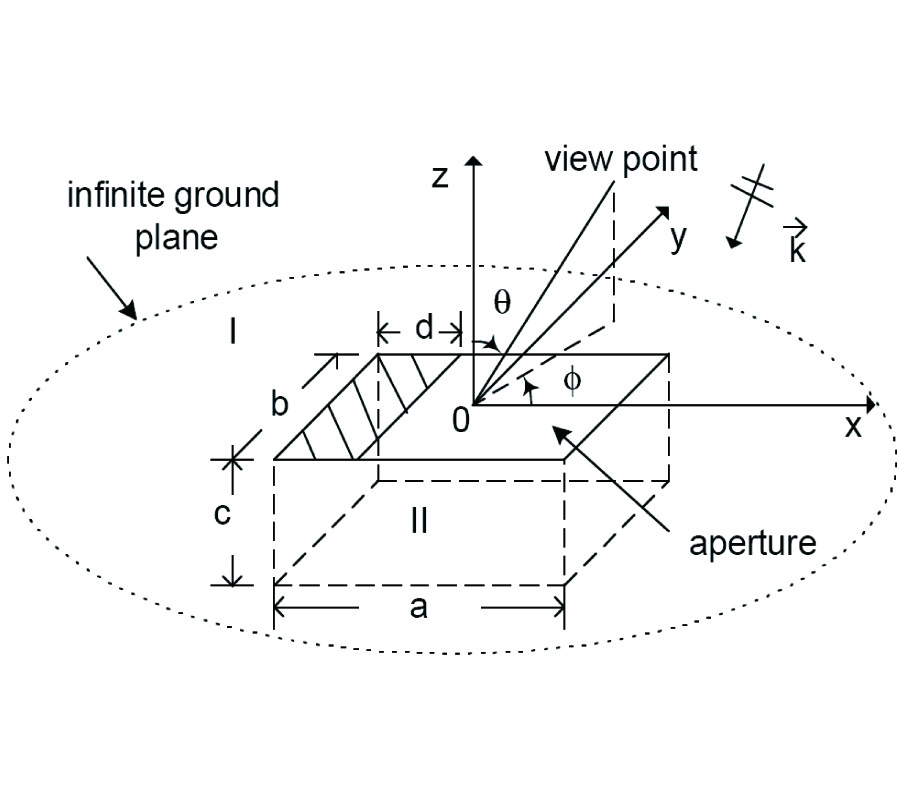Fast Solution for Large Scale Electromagnetic Scattering Problems Using Wavelet Transform and Its Precondition

Xing-Chang Wei and Er Ping Li

Nowadays, electrically large complex electromagnetic problems exist in modern defence and communication industry. Accurate and efficient calculation for such electromagnetic radiation and scattering is a high computational complex task and a challenge to conventional electromagnetic solvers such as Method of Moment (MOM) where high memory and long computational time are required owing to its large size compared to operating wavelength. This paper presents the fast solution method with wavelet transform in the computation of scattering from large scale complex objects. Because of the vanishing moments, the moment matrices arising in these problems are sparsified by wavelet, and consequently, the induced current and equivalent magnetic current can be obtained quickly. Moreover, a precondition method is postulated and implemented in the fast solution of the transformed moment matrix equation with iteration methods.

PIER Vol. 38, 223-252, 2002. doi:10.2528/PIER02042604

###A Spectral-Domain Solution for the Scattering Problem of a Circular Cylinder Buried in a Dielectric Half-Space

Fabrizio Frezza, Lara Pajewski, and Giuseppe Schettini

A spectral-domain solution is employed to completely characterize the two-dimensional electromagnetic plane-wave scattering problem by a perfectly conducting circular cylinder buried in a dielectric half-space. Use is made of the plane-wave spectrum to consider the diffraction, reflection and transmission of cylindrical waves. Suitable adaptive integration techniques are employed to numerically solve the spectral integrals. The method is valid for any value of the cylinder radius, and of the distance between the cylinder and the interface. Numerical results are presented for both near- and far-field cases and for both TM and TE polarizations, and a comparison with other results in the literature is discussed.

PIER Vol. 38, 199-221, 2002. doi:10.2528/PIER02070604

### Non-Relativistic Electromagnetic Scattering: ``Reverse Engineering" Using the Lorentz Force Formulas

Dan Censor

For almost a century, velocity dependent scattering problems are solved in the context of Einstein's Special Relativity theory. Most interesting problems involve non-uniform motion, which is heuristically justified by assuming the validity of the "instantaneous velocity" approximation. The present study attempts to provide a consistent postulational foundation by introducing boundary conditions based on the Lorentz force formulas. The methodology used here is dubbed "reverse engineering": Being aware of the relativistic results, we show that they are replicated, (at least) to the first order in β = v/c by the present method. Specific problems are discussed to demonstrate the power of the method, and pave the way to future research in this problem area. Specifically, by realizing that at the boundary we deal with signals, which are derived from waves, only the latter being subject to the wave equations, it is feasible to apply boundary conditions and construct appropriately the scattered waves in space. It is shown that the present approach is also consistent with the Minkowski constitutive relations which are exploited for solving problems where the medium moves parallel with respect to the boundaries.

PIER Vol. 38, 167-198, 2002. doi:10.2528/PIER02052403

###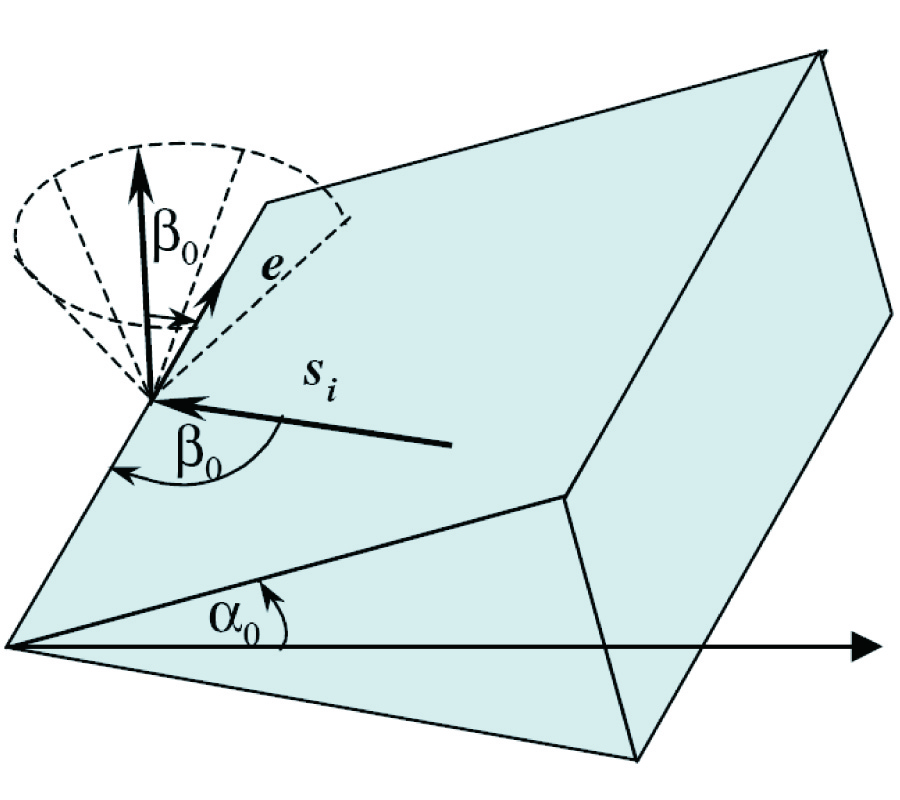Diffraction of a Transverse Electric (TE) X-Wave by Conducting Objects

Ahmed Mohamed Attiya, Amr Shaarawi, and Ioannis Besieris

A study of the diffraction and scattering of a transverse electric X-wave by conducting bodies is presented based on the timedomain, uniform theory of diffraction method and the pulsed plane wave representation of an X-wave. The latter allows the calculation of the diffraction and scattering of each pulsed plane wave component of the incident X-wave at the observation point. The superposition of the individual diffracted and scattered pulsed plane wave components yields the diffracted and scattered field due to an incident X-wave. First, the scattering from a perfectly conducting infinite wedge is studied. Then, the case of a circular conducting disk is considered as an example of a finite scatterer. Numerical results illustrating the effectiveness of the approach, as well as an estimate of the limits of its applicability, are provided.

PIER Vol. 38, 147-166, 2002. doi:10.2528/PIER02052406

###Comparative Analysis Between Conical and Gaussian Profiled Horn Antennas

Ahmed Kishk

A parametric study is performed to the conical and Gaussian profiled horn antennas. Corrugations are added to these horns to further improve their radiation characteristics. The analyses are performed numerically using a body of revolution code, which uses the method of moments. The obtained numerical results are illustrated graphically to show the performance of the horns in terms of phase center, return loss, efficiency with parabolic reflector, directivity, and cross polarization of the horns. Results obtained conclude that the Gaussian profiled horns perform better than the existing conical horn antenna system. The Gaussian profiled horns provide higher efficiency, lower cross polarization, lower sidelobe levels as well as wider bandwidth. The objective of this article is to provide some understanding to the Gaussian profiled horns that might be of help to the new antenna designers.

PIER Vol. 38, 125-146, 2002. doi:10.2528/PIER02080103

###Magnetic Potential Green's Dyadics of Multilayered Waveguide for Spatial Power Combining Applications

Milan Lukic and Alexander Yakovlev

Integral equation formulation and magnetic potential Green's dyadics for multilayered rectangular waveguide are presented for modeling interacting printed antenna arrays used in waveguidebased spatial power combiners. Dyadic Green's functions are obtained as a partial eigenfunction expansion in the form of a double series over the complete system of eigenfunctions of transverse Laplacian operator. In this expansion, one-dimensional characteristic Green's functions along a multilayered waveguide are derived in closed form as the solution of a Sturm-Liouville boundary value problem with appropriate boundary and continuity conditions. A method introduced here is based on the transmission matrix approach, wherein the amplitude coefficients of forward and backward traveling waves in the scattered Green's function in different dielectric layers are obtained as a product of transmission matrices of corresponding layers. Convergence of Green's function components in the source region is illustrated for a specific example of a two-layered, terminated rectangular waveguide.

PIER Vol. 38, 111-123, 2002. doi:10.2528/PIER02081301

### Wave Beam Propagation in Layered Media

Fridrikh G. Bass and L. Resnick

Using rather general assumptions, wave beam propagation is considered in a medium constituted of two half-spaces with smoothly changing properties, these latter changing stepwise at the half-spaces' interface. Expressions for the beam-shape change in the course of propagation are obtained. General results are applied to a Gaussian beam propagating in a series chain, and to fields described by the Helmholtz equation.

PIER Vol. 38, 97-110, 2002. doi:10.2528/PIER02091601

###Analytical Model for Regular Dense Arrays of Planar Dipole Scatterers

Ari Viitanen, Ilari Hanninen, and Sergei Tretyakov

An analytical boundary condition for modeling the electromagnetic properties of planar regular dense arrays of dipole particles for oblique incidence of plane waves is developed.The regular array is assumed to be dense which means that the dipole particles are close to each other.The interaction between the dipole particles is taken into account by interaction constant.The expression for the interaction constant is written in analytical form and is used for developing a transmission-line model for arrays of planar dipole scatterers.The regular dense array is modeled as a shunt impedance which is different for TM and TE polarizations.

PIER Vol. 38, 47-95, 2002. doi:10.2528/PIER02091902

###Surface Green's Function of the Helmholtz Equation in Spherical Coordinates

F. Michael Kahnert, Adrian Doicu, and Jochen Wauer

The surface Green's function belonging to the non-spherical exterior boundary value problem of Helmholtz's equation in spherical coordinates is derived. This is performed in two ways, first by applying the Separation of Variables method, and, second, by using the Method of Lines as a special Finite-Difference technique. With this Green's function we are able to resolve some contradictions concerning conceptual aspects of the Separation of Variables method, the Finite-Difference methods, and the Boundary Integral Equation methods which have been developed for rigorously solving non-separable boundary value problems. The necessary mathematical background, the relation to Waterman's T matrix, and simplifications due to certain symmetry properties of the boundary surface will be discussed. In this paper we focus on the scalar problem. The extension to the vector case for electromagnetic wave scattering is in preparation and will be published later.

PIER Vol. 38, 29-45, 2002. doi:10.2528/PIER02092502

###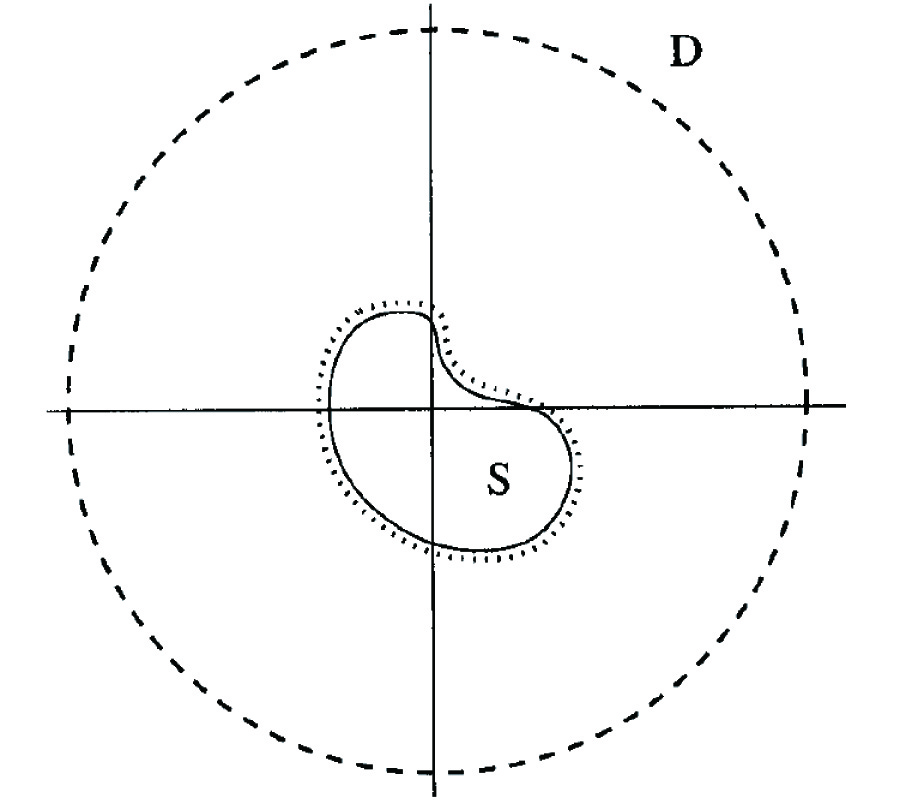Behavior of the Regularized Sampling Inverse Scattering Method at Internal Resonance Frequencies

Karl Warnick

The original proof of the Colton-Kirsch regularized sampling inverse scattering algorithm does not apply at frequencies which are eigenvalues of the interior Helmholtz problem. We explain numerical observations of the behavior of the method and show that useful information about scatterer shape can be obtained at internal resonance frequencies.

PIER Vol. 38, 1-27, 2002. doi:10.2528/PIER02092503

###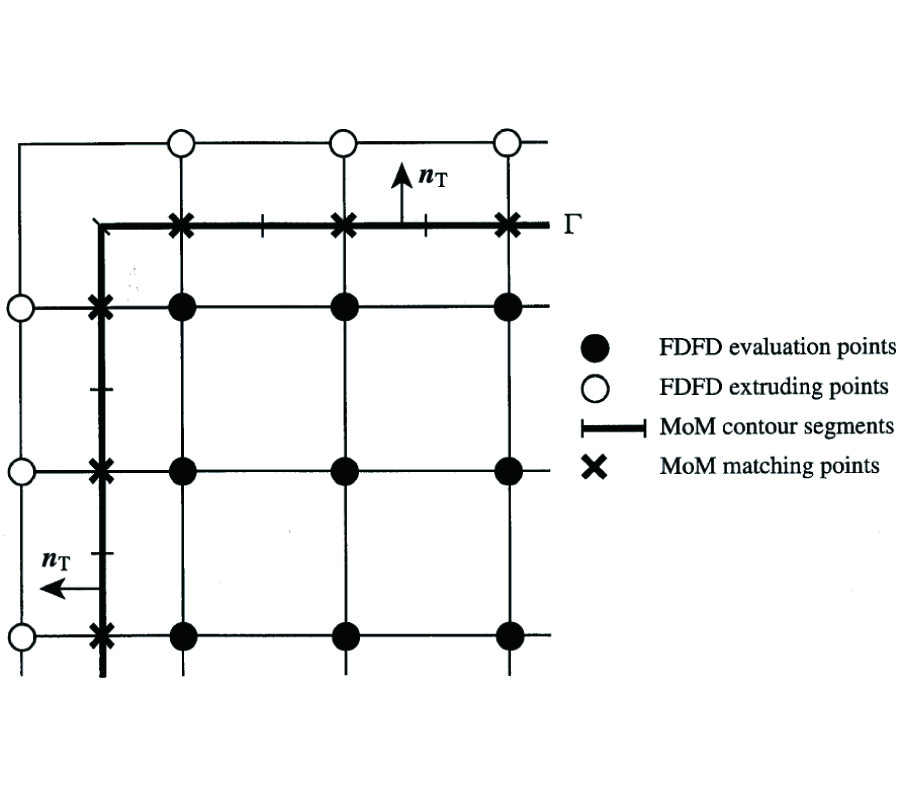A Hybrid Fdfd-Bie Approach to Two-Dimensional Scattering from an Inhomogeneous Biisotropic Cylinder

Martin Norgren

The scattering problem for an inhomogeneous twodimensional biisotropic cylinder is solved in the frequency-domain by means of a hybrid method, in which finite difference equations in the interior region are combined with a mesh truncation in terms of a boundary integral equation that realizes a global absorbing boundary condition. The influences of the chirality and non-reciprocity parameters on the scattering properties are investigated. Numerical results for the bistatic echo widths are presented and compared with a reference solutions in the circular cases and it is found that the method yields more accurate results than what can be achieved with a local absorbing boundary condition. It is realized that, for a given mesh, the method presented is computationally slower than a method based on a local absorbing boundary condition but in on the other hand the method is much faster than the readily used method of moments. The present method is thus suitable for solving scattering problems involving scatterers of intermediate sizes.